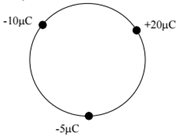# Problem: Three point charges are held on the circumference of a circle of radius 20 cm as shown in the figure. Assume that the electric potential is defined to be zero at infinity. Determine the electric potential at the center of the circle. (a) +6.0 x 104 V (b) +2.3 x 105 V (c) -4.6 x 105 V (d) –7.8 x 105 V (e) zero volts

🤓 Based on our data, we think this question is relevant for Professor Schulte's class at UCF.

###### Problem Details

Three point charges are held on the circumference of a circle of radius 20 cm as shown in the figure. Assume that the electric potential is defined to be zero at infinity. Determine the electric potential at the center of the circle.

(a) +6.0 x 10V

(b) +2.3 x 10V

(c) -4.6 x 10V

(d) –7.8 x 10V

(e) zero volts